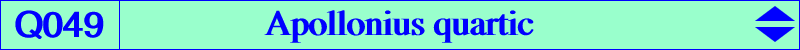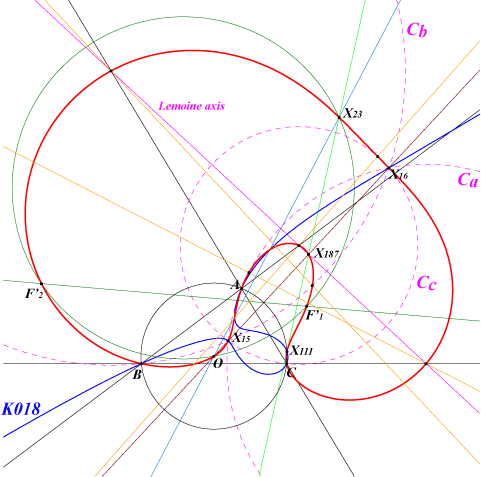too complicated to be written here. Click on the link to download a text file.X(3), X(15), X(16), X(23), X(111), X(187), X(5000), X(5001), X(6104), X(6105) inversive images in the circumcircle of X(13), X(14), X(368), foci of the Steiner in-ellipse, vertices of the fourth Brocard triangle centers of Apollonian circles (inversive images in the circumcircle of the vertices of the second Brocard triangle)Let P be a point and Pa, Pb, Pc its inversive images in the Apollonian circles C_a, C_b, C_c respectively. ABC and PaPbPc are perspective if and only if P lies on the circumcircle or on the Apollonius quartic Q049. The inversive image of Q049 in the circumcircle is K018, the second Brocard cubic. Q049 is a bicircular quartic.